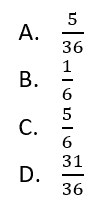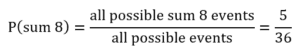# PCAT Quantitative Reasoning – Two Dice Probability

Two 6-sided dice are rolled simultaneously. What is the probability that their sum will not be 8?##### Explanation

D is correct. Recall that the probability of an event occurring is equal to the number of possible outcomes where that event occurs divided by the total number of all possible outcomes. The probability of an event not occurring is equal to 1 minus the probability of that event occurring.First, let’s determine the total number of possible events that could occur. There are six possible outcomes from rolling Die 1, and there are six possible outcomes from rolling Die. The probability of two simultaneous independent events—such as one outcome occurring from Die 1 and another outcome occurring from Die 2—is the product of their probabilities. Likewise, the total number of outcomes that could occur is the product of the number of outcomes associated with each die:

6×6=36 possible outcomes

Now that we’ve determined the total number of possible outcomes, let’s determine how may possible outcomes result in a sum of 8. For the PCAT, the most efficient way to do this is to create a table that lists all the possibilities that could result of a sum of 8:Therefore, there are 5 possible events that would generate the outcome of interest, a sum of 8. The probability that simultaneously rolling two dice will produce a sum of 8 must be:This tells us that the probability of rolling a sum of 8 is 5/36. The probability of not rolling a sum of 8—of rolling anything else—must be:#### Submit a Comment

This site uses Akismet to reduce spam. Learn how your comment data is processed.